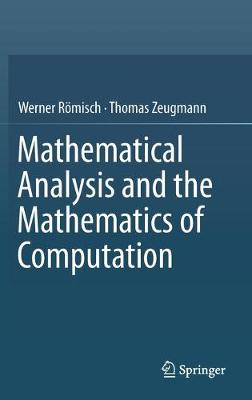•Mathematical Analysis and the Mathematics of Computation (Hardback)

(author), (author)
£74.99
Hardback 703 Pages / Published: 13/10/2016
• We can order this

Usually dispatched within 3 weeks

This book is a comprehensive, unifying introduction to the field of mathematical analysis and the mathematics of computing. It develops the relevant theory at a modern level and it directly relates modern mathematical ideas to their diverse applications.

The authors develop the whole theory. Starting with a simple axiom system for the real numbers, they then lay the foundations, developing the theory, exemplifying where it's applicable, in turn motivating further development of the theory. They progress from sets, structures, and numbers to metric spaces, continuous functions in metric spaces, linear normed spaces and linear mappings; and then differential calculus and its applications, the integral calculus, the gamma function, and linear integral operators. They then present important aspects of approximation theory, including numerical integration. The remaining parts of the book are devoted to ordinary differential equations, the discretization of operator equations, and numerical solutions of ordinary differential equations.

This textbook contains many exercises of varying degrees of difficulty, suitable for self-study, and at the end of each chapter the authors present more advanced problems that shed light on interesting features, suitable for classroom seminars or study groups. It will be valuable for undergraduate and graduate students in mathematics, computer science, and related fields such as engineering. This is a rich field that has experienced enormous development in recent decades, and the book will also act as a reference for graduate students and practitioners who require a deeper understanding of the methodologies, techniques, and foundations.

Publisher: Springer International Publishing AG
ISBN: 9783319427539
Number of pages: 703
Weight: 1585 g
Dimensions: 235 x 155 x 40 mm
Edition: 1st ed. 2016

MEDIA REVIEWS

"This textbook is mainly based on lectures, seminars and compositions read, taught and made by the authors at Humboldt University in Berlin and is intended as a four semester course. ... this is a well-written textbook and I recommend it for undergraduate, graduate and Ph.D students in mathematics, computer science, engineering and other related fields." (Sorin Gheorghe Gal, Mathematical Reviews, June, 2017)

"Roemisch and Zeugmann's Mathematical analysis and the mathematics of computation is a lucid introductory textbook that unites mathematical analysis with the mathematics of computation in a single volume. ... It has many exercises and additional problems in each chapter to enhance the problem-solving ability of the student. ... readership includes undergraduate and graduate students of mathematics, computing, and related fields such as engineering. It should also serve as useful reference material for others, given the continuous progress in this field." (Computing Reviews, June, 2017)

"The book is written in a nicely readable style. It comes with plenty of carefully designed figures where color has been used in a well-thought-out way. Moreover, the text contains a large number of problems and exercises and many remarks describing the historical developments that have led to the current state of the art. I fully recommend it to be used by (beginning and advanced) students as material for self-study or by lecturers as a textbook." (Kai Diethelm, Computing Reviews, May, 2017)

"This is a text for a first real analysis course. Its selling point is that it presents a wide variety of topics with a uniform notation and terminology, and the same level of abstraction. It also presents an integrated view of approximation and mathematical analysis. ... I admire its goals and like its execution ... ." (Allen Stenger, MAA Reviews, maa.org, March, 2017)

"The authors keep in touch with the necessities of undergraduate students by providing the theory of infinite series, the differential calculus in R and Rn, power series and elementary functions. ... The material of the book is presented in a clear way with precise and understandable worked out proofs. ... for computer science students the topics of the book go well beyond what they normally are willing to learn and also what they will need in their carreer." (Rolf Dieter Grigorieff, zbMATH 1358.65001, 2017)